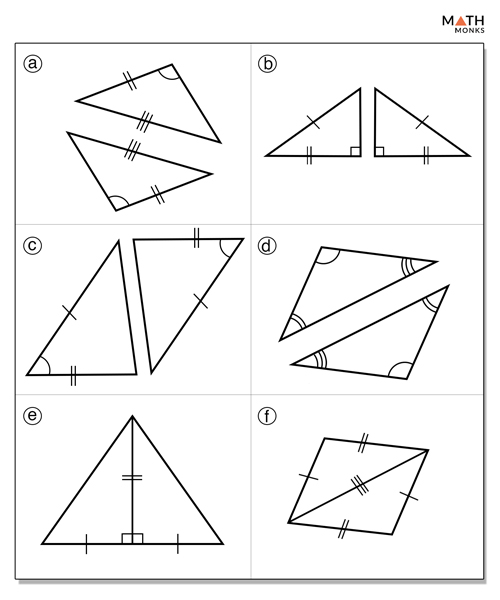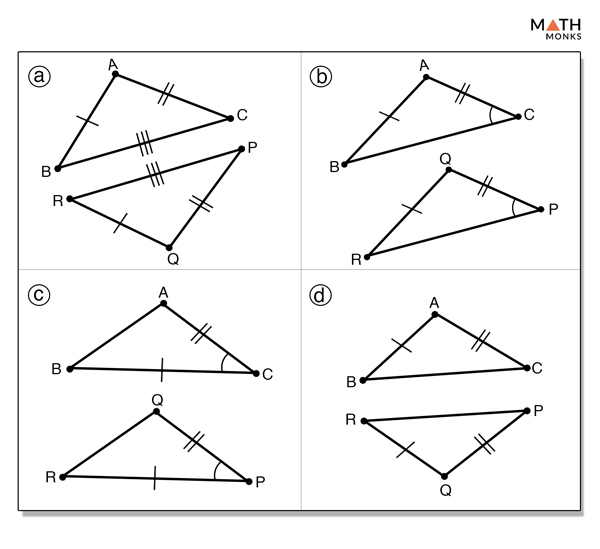# Congruent Triangles

The word ‘congruent’ is used to describe objects that have the same shape or dimension. Congruence is the term used to define an object and its mirror image. Two or more objects are said to be congruent if they superimpose on each other or in other words they are of same shape and size. This property of being congruent is called congruency.

## What are Congruent Triangles

Congruent triangles are triangles having all three sides of exactly the same length and all three angles of exactly the same measure. Thus, congruent triangles are mirror image of each other. On the other hand, triangles that are not congruent are called non-congruent triangles. In triangles, the abbreviation CPCT – Corresponding Parts of Congruent Triangles is used to show congruency.

Shown below are two triangles, ΔABC and ΔEFG that are congruent, which is mathematically represented as ΔABC ≅ ΔEFG. Their corresponding parts are shown below.

Thus, the corresponding parts of congruent triangles are congruent.

## Properties

Triangles can become congruent through flip (reflection), turn (rotation), or side-wise movement (translation) as shown below. It means, one triangle can be congruent to the other although their equal sides and angles are not in the same position.

1) Reflexive Property

It states that the mirror image of any triangle is always congruent to it.

2) Symmetric Property

It states that the congruence works in both frontward and backward directions such that a triangle formed by rotation is always congruent to the other.

3) Transitive Property

It states that if two triangles are congruent to a third triangle, they are also congruent to each other.

## Proving Triangles Congruency: Rules & Theorems

Triangle congruence is a set of rules or measures used to prove if two or more triangles are congruent. The five ways of identifying congruent triangles are shown below.

### 1) Side–Side-Side (SSS) Theorem

It states that if three sides of one triangle are congruent to three sides of another triangle, then the two triangles are congruent.

### 2) Side–Angle–Side (SAS) Theorem

It states that if two sides and the included angle of one triangle are congruent to two sides and included angle of another triangle, then the two triangles are congruent.

### 3) Angle–Side–Angle (ASA) Theorem

It states that if two angles and the included side of one triangle are congruent to two angles and included side of another triangle, then the two triangles are congruent.

### 4) Angle–Angle–Side (AAS) Theorem

It states that if two angles and a non-included side of one triangle are congruent to two angles and a non-included side of another triangle, then the two triangles are congruent.

### 5) Hypotenuse-Leg (HL) Rule of a Right Angle Theorem

It states that if the hypotenuse and one leg of a right triangle are congruent to the hypotenuse and one leg of another right triangle, then the two triangles are congruent.

### Theorems Not Applicable to Prove Triangles Congruency

AAA Rule: If all the corresponding angles of a triangle are congruent with the corresponding angles of another triangle, the triangles will be of the same shape but not necessarily of the same size. Thus, they will not be congruent.

SSA Rule: If two corresponding sides and an angle are congruent, the said triangles may not be congruent as there are two different shapes possible for the triangle.

## Congruent vs Similar Triangles

As we know, congruent triangles are triangles having the same shape and the same size. Mathematically, triangles with same side length and angle measure are called congruent triangles. In contrast, triangles having same shape but of different size are called similar triangles.

Thus, all congruent triangles are similar but all similar triangles are not congruent.

## Solved ExamplesBy what method would each of the given triangles be proven congruent?

Solution:

(a) None, as there is no SSA method, (b) HL, (c) SAS, (d) None, as there is no AAA method, (e) SAS, and (f) SSS, the third pair of congruent sides is shared by the two triangles.Which of the following pairs of triangles can be proven congruent by SAS?

Solution:

(c), as BC ≅ PR, ∠ACB ≅ ∠QPR, and AC ≅ PQWhich of the following pairs of triangles are congruent by ASA?

Solution:

(c), as∠OAB ≅ ∠ODC (given), AB ≅ CD (given), and ∠AOB ≅ ∠COD (vertically opposite angles).

## FAQs

Q1. What triangle has no congruent sides?

Ans. Scalene triangle.

Q2. What triangle has three congruent sides?

Ans. Equilateral triangle.

Q3. What triangle has two congruent sides?

Ans. Isosceles triangle.

Q4. What is a triangle with at least two congruent sides called?

Ans. Isosceles triangle.

Q5. What is a triangle with one right angle and no congruent sides called?

Ans. Right scalene triangle.

Q6. What is a triangle with three acute angles and three congruent sides called?

Ans. Equilateral triangle.

Q7. Which rigid motion verifies the triangles are congruent by SAS?

Ans. Reflection.

Q8. Are all equilateral triangles congruent?

Ans. Since each angle in an equilateral triangle measures 60° and all sides are of the same length, all equilateral triangles are congruent.

Q9. Are all right triangles congruent?

Ans. No, right triangles are congruent if both the hypotenuse and one leg are of the same length.

Q10. How many congruent sides does an equilateral triangle have?

Ans. Equilateral triangles have three congruent sides.

Q11. What is a triangle with three acute angles and no congruent sides?

Ans. Acute scalene triangle.

• More Resources